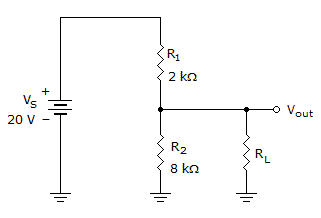# Electronics - Series-Parallel Circuits - Discussion

### Discussion :: Series-Parallel Circuits - General Questions (Q.No.13)

13.If the load in the given circuit is open, what is the unloaded output voltage?

 [A]. 0 V [B]. 4 V [C]. 16 V [D]. 20 V

Explanation:

No answer description available for this question.

 Divya said: (Sep 15, 2011) Unloaded o/p volt. means rl=0 Therefore r1&r2 are in series i=v/r=20/(2k+8k)=2mA v across r2 is v=ir=2m*8k=16.

 Manoj Bhat said: (Apr 24, 2015) Find the current in the circuit: Add R1 & R2 which are in series. R1+R2 = 10 kohm. Current, I = 2 m amps. Since vout is taken, V = IR1. V = 2m*2k. V = 4V. Therefore Vout = 20-4 = 16V.